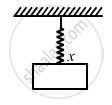Department of Pre-University Education, KarnatakaPUC Karnataka Science Class 11
Advertisement Remove all ads

# A Block Suspended from a Vertical Spring is in Equilibrium. Show that the Extension of the Spring Equals the Length of an Equivalent Simple Pendulum - Physics

Sum

A block suspended from a vertical spring is in equilibrium. Show that the extension of the spring equals the length of an equivalent simple pendulum, i.e., a pendulum having frequency same as that of the block.

Advertisement Remove all ads

#### Solution

An equivalent simple pendulum has same time period as that of the spring mass system.
The time period of a simple pendulum is given by,

$T_p = 2\pi\sqrt{\left( \frac{l}{g} \right)}$

where l is the length of the pendulum, and
g is acceleration due to gravity.

Time period of the spring is given by,

$T_s = 2\pi\sqrt{\left( \frac{m}{k} \right)}$

where is the mass, and
is the spring constant.

Let x be the extension of the spring.
For small frequency, TP ​can be taken as equal to TS.$\Rightarrow \sqrt{\left( \frac{l}{g} \right)} = \sqrt{\left( \frac{m}{k} \right)}$

$\Rightarrow \left( \frac{l}{g} \right) = \left( \frac{m}{k} \right)$

$\Rightarrow l = \frac{mg}{k} = \frac{F}{k} = x$

($\because$ restoring force = weight = mg

$\therefore$ l = x (proved)

Is there an error in this question or solution?
Advertisement Remove all ads

#### APPEARS IN

HC Verma Class 11, 12 Concepts of Physics 1
Chapter 12 Simple Harmonics Motion
Q 10 | Page 252
Advertisement Remove all ads
Advertisement Remove all ads
Share
Notifications

View all notifications

Forgot password?